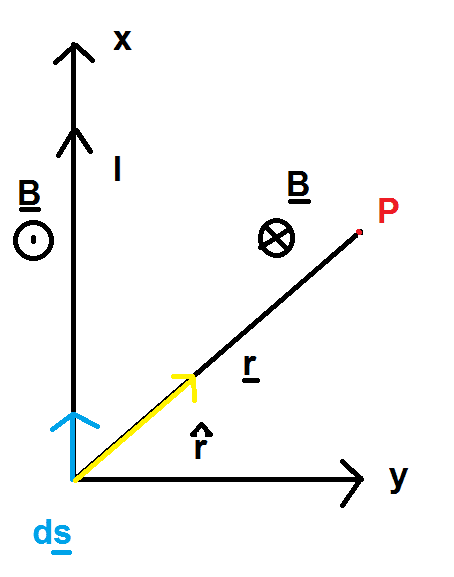# Deriving Biot-Savart's Law, kinda a math problem not really physics

## Homework Statement

Basically I am deriving Biot-Savart's Law using the vector method. The one my book gives me is impossible to remember

## The Attempt at a Solution

http://img39.imageshack.us/img39/6584/pictureson.th.png [Broken]

Uploaded with ImageShack.us

So I am actually just going to do the indefinite integral first, but I got so many differentials, it's ridiculous

$$d\vec{s} = <0,d\vec{y},0>$$

$$\hat{r} = <\sin\theta,\cos\theta,0>$$

$$\begin{vmatrix} i & j & k\\ 0& d\vec{y} &0 \\ \sin\theta & \cos\theta &0 \end{vmatrix} = -\sin\theta d\vec{y} \hat{k}$$

So now my integral is

$$\vec{B} = \frac{\mu_0 I}{4\pi}\int \frac{-\sin\theta d\vec{y} \hat{k}}{r^2}$$

Now here is the problem, I had trouble finding what r2 is, I decided to let it be $$x^2 + y^2 = r^2$$

But then my integral becomes

$$\vec{B} = \frac{\mu_0 I}{4\pi} \int \frac{-\sin\theta d\vec{y} \hat{k}}{x^2 + y^2}$$

Now the problem is, I could do a trig substitution and let y = xtanθ

$$\vec{B} = \frac{\mu_0 I}{4\pi} \int \frac{-\sin\theta d\vec{y} \hat{k}}{x^2 + x^2 tan\theta ^2}$$

$$\vec{B} = \frac{\mu_0 I}{4\pi} \int \frac{-\sin\theta d\vec{y} \hat{k}}{x^2 sec\theta ^2}$$

$$\vec{B} = \frac{\mu_0 I}{4\pi} \int \frac{-\sin\theta \cos^2\theta d\vec{y} \hat{k}}{x^2 }$$

Now the question is, how do I get rid of the x2?

EDIT: TEX FIXED

Last edited by a moderator:

## Answers and Replies

Please help me, it is driving me insane that I can't solve this

Here is another attempt

$$\vec{B} = \frac{\mu_0 I}{4\pi} \int \frac{-cos\theta d\vec{y} \hat{k}}{x^2 + y^2}$$

I drew my triangle again and I substitute $$cos\theta = \frac{y}{\sqrt{x^2 + y^2}}$$

$$\vec{B} = \frac{\mu_0 I}{4\pi} \int \frac{-\frac{y}{\sqrt{x^2 + y^2}}d\vec{y} \hat{k}}{x^2 + y^2}$$

$$\vec{B} = \frac{\mu_0 I}{4\pi} \int \frac{-y d\vec{y} \hat{k}}{(x^2 + y^2)^\frac{3}{2}}$$

So I ran this on Mathematica setting my bounds from -inf to +inf and I got 0...which is bad...very bad

Last edited:
Please someone, Sammy S, gneil, anyone please lol

jhae2.718
Gold Member
What are you using as your coordinate system? (The magnitude of a x b should be |a||b|sin(theta), and you have a cos(theta)...)

I know, my x is your standard "y" in this picture and vice-versa

jhae2.718
Gold Member
Is this the way it is:Edit: with theta between the x axis and r...

jhae2.718
Gold Member
I'm not quite sure how you've setup your coordinate system, but it might be easier if you define some angle phi = pi/2 - theta and integrate in terms of phi...

(Of course, if the wire is infinite, the easiest way is to use Ampere's law...)

I'm not quite sure how you've setup your coordinate system, but it might be easier if you define some angle phi = pi/2 - theta and integrate in terms of phi...

(Of course, if the wire is infinite, the easiest way is to use Ampere's law...)

No I already know how it was derived. I know what you are getting at because that's the one the book used. But I didn't like it because it was too difficult to remember. This one I find much more intuitive. No Ampere's law please

Which part of the coordinate are you confused about?

jhae2.718
Gold Member
Just to let you know, I haven't forgotten about this, but I'm busy for a little bit...

It would help if you drew a coordinate axes on the drawing; I'm trying to use the same coordinate system as you are.

SammyS
Staff Emeritus
Science Advisor
Homework Helper
Gold Member
If you are deriving the Boit-Savart Law, what is it you are starting with?

The general equation?

$$\vec{B} = \frac{\mu_0 I}{4\pi} \int \frac{\vec{ds} \times \hat{r}}{r^2}$$

SammyS
Staff Emeritus
Science Advisor
Homework Helper
Gold Member
The general equation?

$$\vec{B} = \frac{\mu_0 I}{4\pi} \int \frac{\vec{ds} \times \hat{r}}{r^2}$$

This is one form of the Biot-Savart Law.

Are you just trying to put it in some form you find easier to work with?

Yeah basically.

This is one form of the Biot-Savart Law.

Are you just trying to put it in some form you find easier to work with?

And to derive it, right now that's what I am doing for a rod

jhae2.718
Gold Member
Are you doing a finite or infinite wire?

Either, I want to get the indefinite integral solved first

jhae2.718
Gold Member
From your first post, I believe your r-hat should be $\hat{r} = \langle \sin(\theta), \cos(\theta), 0 \rangle$.

SammyS
Staff Emeritus
Science Advisor
Homework Helper
Gold Member
What can you possibly mean by: $$d\vec{y}\hat{k}$$ in your integral?

Mathematica may have interpreted that as a dot product of those two vectors, which does make zero the answer.

No I left out $$\hat{k}$$

I just typed in int{y/(y^2 + x^2)^(3/2),y}

From your first post, I believe your r-hat should be $\hat{r} = \langle \sin(\theta), \cos(\theta), 0 \rangle$.

No from the way I defined x and y, it is different

jhae2.718
Gold Member
Because I get the correct answer if I use that r-hat.

The way you have your angle defined, x = rsin(theta) and y = rcos(theta)...